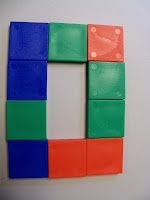## Monday, April 11, 2016

### Perimeter

Today we began perimeter. The children learned perimeter last year, so we just briefly discussed what it is. "Peri" means around, and "meter" means measure. Therefore, perimeter is simply measuring around an object. After we practiced finding the perimeter of several rectangles and squares, I asked the kids to make me a shape using color tiles with a perimeter of 10. Out of all 40 math students I had yesterday, only one did it correctly. Almost every child used 10 tiles to make a rectangle, as pictured. I let them finish, then we discussed why this wasn't correct. "You all told me the perimeter means the distance around the outside. Then you  made me a shape where the distance around was 14. Why does using 10 tiles give you a perimeter other than 10?"  Eventually the kids were able to explain the tiles would all have to have only one side facing out to give them a perimeter of 10, which isn't possible. Then I challenged the kids to go back and fix their shapes. All were able to do it correctly. We also looked at three different shapes (also pictured) that used a different number of tiles, but had a perimeter of 10. Again, we focused on the sides facing out and discussed how this was possible.

After this, we made shapes with a perimeter of 12, then 20. All were able to do this correctly, and many got very creative with their shapes!Incorrect Perimeter of 10How do these all have a perimeter of 10?Area vs. Perimeter Anchor Chart